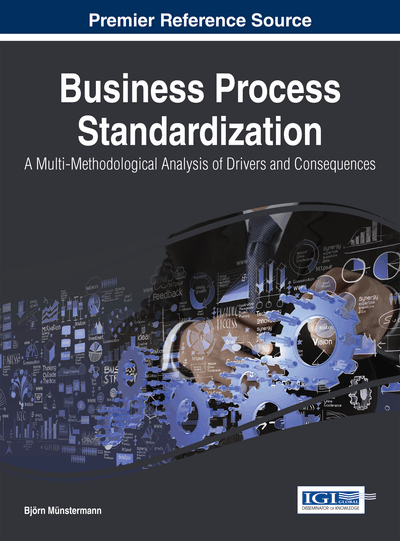# Evaluation of BPS and Its Impact: Quantitative Approach

DOI: 10.4018/978-1-4666-7236-9.ch005

## Abstract

The goal of this chapter is a quantitative analysis of the research models and the research hypotheses developed in Chapter 4. In a first step, Structural Equation Modeling (SEM) using the Partial Least Squares (PLS) methodology as applied in this research and a set of quality criteria to evaluate PLS models are introduced. Then, the surveys conducted—“Recruiting Trends 2007 and 2009”—are presented with details on the process in focus, the construct operationalizations used, as well as the data collection and evaluation carried out. Finally, the results of the survey are presented.
Chapter Preview
Top

## 5.1 Survey Research Method And Research Design

### 5.1.1 Structural Equation Modeling (SEM) Using the Partial Least Squares (PLS) Methodology

Structural Equation Modeling (SEM) is a statistical technique applied in many disciplines for testing and estimating causal relations. SEM techniques are considered as the second generation of multivariate analysis and offer researchers “the ability to perform path-analytic modeling with latent variables” to typically test a set of hypotheses empirically (Chin & Newsted, 1999, p. 307).

Compared to first generation techniques of multivariate analysis (e.g. discriminant analysis, multiple regression, or classical analysis of variance and covariance) the SEM approach leads to better results as according to Chin and Newsted they “involve generalizations and extensions of first generation procedures” (Chin & Newsted, 1999, p. 308).

That SEM is the best available approach for testing path diagrams empirically, especially when these involve latent variables with multiple indicators, is underlined by a recent article of Gefen, Rigdon, and Straub (2011). The authors write that “there are unique advantages to SEM over linear regression” and that this makes “SEM a priori the method of choice in analyzing path diagrams when these involve latent variables with multiple indicators” (Gefen et al., 2011, p. iv). For a more detailed discussion on SEM we refer the reader to publications such as Gefen, Straub, and Boudreau (2000), Chin (2010) or Joachim (2012) from which (chapter 3.3) we adopted parts of this sub-section.

A theoretical construct that cannot be measured directly is called a “latent variable” (sometimes also referred to as “construct” or “dependent/independent variable”) in SEM. To measure the unobservable latent variable a measurement instrument consisting of indicators (resp. observed/manifest variables) has to be developed. In most cases the indicators correspond to specific items (questions or statements) of a questionnaire.

There are two types of measurement instruments that can be differentiated, “reflective” and “formative” measurement models (Albers & Hildebrandt, 2006; Bagozzi, 2011; Bollen & Bauldry, 2011; Jarvis, MacKenzie, & Podsakoff, 2003; MacKenzie, Podsakoff, & Jarvis, 2005). In a reflective measurement model the indicators are formulated to “reflect” the latent variable; i.e. if the value of the construct changes, all indicators are supposed to change accordingly. In a formative measurement model, the indicators “form” the construct; i.e. if one of the indicators changes, the latent variable is supposed to change accordingly. The indicators belonging to a reflective measurement model should statistically correlate highly with each other while, to avoid multi-collinearity issues, the indicators of a formative measurement model should not. In this book, we operationalized all constructs by reflective multi-item measures.

Constructs, that consist of only one latent variable with a corresponding measurement model, are called “first-order constructs”. Besides first-order constructs also “second-order constructs”, that consist of two or more first-order constructs are widely used. Typically, these second- or higher order latent variables are used whenever a theoretical construct comprises multiple sub-dimensions which each should be measured with a corresponding own measurement model (Chin, 1998a; Polites, Roberts, & Thatcher, 2012).

Typically, SEM models comprise two parts: the “measurement model” (also called “outer model”) and the “structural model” (also called “inner model”). The measurement (or outer) model contains and describes the relationships of the latent variables with their respective indicators. The structural (or inner) model comprises the hypothesized causal paths between the latent variables and thereby represents the relationships between the latent variables to be tested.

As a SEM combines a measurement and a structural model to be simultaneously assessed and is able to “analyze many stages of independent and dependent variables […] into one unified model” it “allows a better estimation of both measurement and structural relationships” (Gefen et al., 2011, p. iv).

In IS research, Partial Least Squares (PLS) and Covariance-Based Structural Equation Modeling (CBSEM) are “the two most widely used types of SEM” (Gefen et al., 2011, p. v). These two types differ with respect to their approach (PLS is variance based while CBSEM is covariance based) and their objective (PLS is rather prediction oriented while CBSEM is rather parameter oriented) (Chin & Newsted, 1999; Gefen et al., 2011). Widely used software packages based on CBSEM are LISREL and AMOS; the PLS approach is, for example, implemented in SmartPLS (Ringle, Wende, & Will, 2005) and PLS-Graph.

For our research we decided to apply the PLS approach for the following reasons:

• PLS is better suited to research where the phenomenon investigated is relatively new and the theoretical models and measures are not well formed and validated in prior research (Chin & Newsted, 1999; Gefen et al., 2011). In addition, it can be a “powerful method of analysis because of minimal demands on measurement scales” (Chin, 1998b, p. 295).

• According to Chin and Newsted (1999) PLS can be specially helpful with small sample sizes.

• PLS provides very good results even if the data underlying indicators used is not normally distributed (Chin, 1998b).

## Complete Chapter List

Search this Book:
Reset